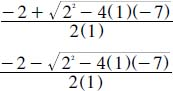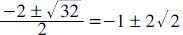# Can all quadratic equations be solved by factoring?

Don’t be fooled: Not all quadratic equations can be solved by factoring. For example, x2 - 3x = 3 is not solvable with this method. One way to solve quadratic equations is by completing the square; still another method is to graph the solution (a quadratic graph forms a parabola—a U-shaped line seen on the graph). But one of the most well-known ways is by using the quadratic formula (see below).

For example, if we want to find the roots of the polynomial x2 + 2x - 7, we can replace the “corresponding” numbers from the initial equation into the quadratic equation; thus, a = 1, b = 2, and c = -7. Substituting these numbers into the quadratic formula, we solve for:That equalsClose

This is a web preview of the "The Handy Math Answer Book" app. Many features only work on your mobile device. If you like what you see, we hope you will consider buying. Get the App